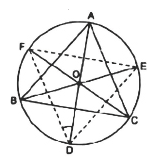Guru

# Bisectors of angles A, B and C of a triangle ABC intersect its circumcircle at D, E and F respectively. Prove that the angles of the triangle DEF are 90°–(½)A, 90°–(½)B and 90°–(½)C. Q.8

• 0

What are the different way for solving the question for class 9th question of circle chapter of math of question no.8 of math please suggest me the best way for solving this question of exercise 10.6 Bisectors of angles A, B and C of a triangle ABC intersect its circumcircle at D, E and F respectively. Prove that the angles of the triangle DEF are 90°–(½)A, 90°–(½)B and 90°–(½)C.

Share

1. Consider the following diagramHere, ABC is inscribed in a circle with center O and the bisectors of ∠A, ∠B and ∠C intersect the circumcircle at D, E and F respectively.

Now, join DE, EF and FD

As angles in the same segment are equal, so,

∠FDA = ∠FCA ————-(i)

∠FDA = ∠EBA ————-(i)

By adding equations (i) and (ii) we get,

∠FDA+∠EDA = ∠FCA+∠EBA

Or, ∠FDE = ∠FCA+∠EBA = (½)∠C+(½)∠B

We know, ∠A +∠B+∠C = 180°

So, ∠FDE = (½)[∠C+∠B] = (½)[180°-∠A]

∠FDE = [90-(∠A/2)]

In a similar way,

∠FED = [90° -(∠B/2)] °

And,

∠EFD = [90° -(∠C/2)] °

• 0